## How to Calculate and Solve for Jaw Crusher Rating | Mineral Processing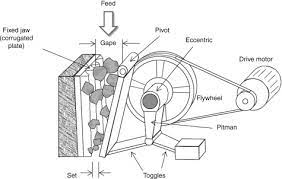The image above represents jaw crusher rating.

To compute for jaw crusher rating, two essential parameters are needed and these parameters are Gape Size (G) and Width of Crusher (W).

The formula for calculating the jaw crusher rating:

Jr = GW

Where:

Jr = Jaw Crusher Rating
G = Gape Size
W = Width of Crusher

Let’s solve an example;
Find the jaw crusher rating when the gape size is 15 and the width of crusher is 5.

This implies that;

G = Gape Size = 15
W = Width of Crusher = 5

Jr = GW
Jr = (15)(5)
Jr = 75

Therefore, the jaw crusher rating is 75.

Calculating the Gape Size when the Jaw Crusher Rating and the Width of Crusher is Given.

G = Jr / W

Where:

G = Gape Size
Jr = Jaw Crusher Rating
W = Width of Crusher

Let’s solve an example;
Find the gape size when the jaw crusher rating is 32 and the width of crusher is 2.

This implies that;

Jr = Jaw Crusher Rating = 32
W = Width of Crusher = 2

G = Jr / W
G = 32 / 2
G = 16

Therefore, the gape size is 16.

## How to Calculate and Solve for Passing Reduction Ratio | Mineral Processing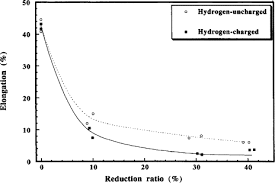The image above represents passing reduction ratio.

To compute for passing reduction ratio, two essential parameters are needed and these parameters are 80% Passing Size of Feed (F80) and 80% Passing Size of Product (P80).

The formula for calculating passing reduction ratio:

Pr = F80 / P80

Where:

Pr = Passing Reduction Ratio
F80 = 80% Passing Size of Feed
P80 = 80% Passing Size of Product

Let’s solve an example;
Find the passing reduction ratio when the passing size of feed is 21 and the passing size of product is 3.

This implies that;

F80 = 80% Passing Size of Feed = 21
P80 = 80% Passing Size of Product = 3

Pr = F80 / P80
Pr = 21 / 3
Pr = 7

Therefore, the passing reduction ratio is 7.

Calculating the Passing Size of Feed when the Passing Reduction Ratio and the Passing Size of Product is Given.

F80 = Pr x P80

Where:

F80 = 80% Passing Size of Feed
Pr = Passing Reduction Ratio
P80 = 80% Passing Size of Product

Let’s solve an example;
Find the passing size of feed when the passing reduction ratio is 3 and the passing size of product is 9.

This implies that;

Pr = Passing Reduction Ratio = 3
P80 = 80% Passing Size of Product = 9

F80 = Pr x P80
F80 = 3 x 9
F80 = 27

Therefore, the passing size of feed is 27.

## How to Calculate and Solve for Crusher Reduction Ratio | Mineral Processing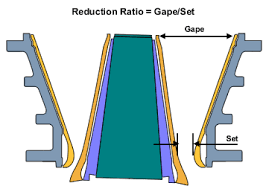The image above represents crusher reduction ratio.

To compute for crusher reduction ratio, two essential parameters are needed and these parameters are Gape Size (G) and Set Size (S).

The formula for calculating crusher reduction ratio:

Rr = G / S

Where:

Rr = Crusher Reduction Ratio
G = Gape Size
S = Set Size

Let’s solve an example;
Find the crusher reduction ratio when the gape size is 20 and the set size is 10.

This implies that;

G = Gape Size = 20
S = Set Size = 10

Rr = G / S
Rr = 20 / 10
Rr = 2

Therefore, the crusher reduction ratio is 2.

Calculating the Gape Size when the Crusher Reduction Ratio and the Set Size is Given.

G = Rr x S

Where:

G = Gape Size
Rr = Crusher Reduction Ratio
S = Set Size

Let’s solve an example;
Find the gape size when the crusher reduction ratio is 8 and the set size is 4.

This implies that;

Rr = Crusher Reduction Ratio = 8
S = Set Size = 4

G = Rr x S
G = 8 x 4
G = 32

Therefore, the gape size is 32.

## How to Calculate and Solve for Specific Surface Area | Mineral Processing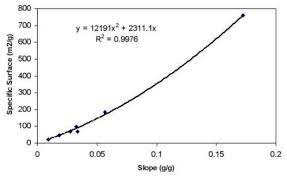The image above represents specific surface area.

To compute for specific surface area, two essential parameters are needed and these parameters are Proportionality Constant (KL) and Size of Particles (D).

The formula for calculating specific surface area:

A = KL/D

Where:

A = Specific Surface Area
KL = Proportionality Constant
D = Size of Particle

Let’s solve an example;
Find the specific surface area when the proportionality constant is 25 and the size of particle is 5.

This implies that;

KL = Proportionality Constant = 25
D = Size of Particle = 5

A = KL/D
A = 25/5
A = 5

Therefore, the specific surface area is 5 m².

Calculating the Proportionality Constant when the Specific Surface Area and the Size of Particle is Given.

KL = A x D

Where:

KL = Proportionality Constant
A = Specific Surface Area
D = Size of Particle

Let’s solve an example;
Find the proportionality constant when the specific surface area is 10 and the size of particle is 4.

This implies that;

A = Specific Surface Area = 10
D = Size of Particle = 4

KL = A x D
KL = 10 x 4
KL = 40

Therefore, the proportionality constant is 40.

## How to Calculate and Solve for Power of Comminution walls | Mineral Processing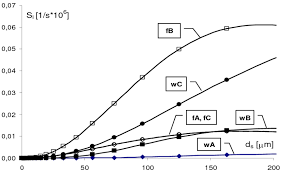The image above represents power of comminution walls.

To compute for power of comminution walls, two essentials parameters are needed and these parameters are Energy (E) and Throughput (T).

The formula for calculating power of comminution walls:

W = E / T

Where:

W = Power of Comminution Walls
E = Energy
T = Throughput

Let’s solve an example;
Find the power of comminution walls when the energy is 15 and the throughput is 10.

This implies that;

E = Energy = 15
T = Throughput = 10

W = E / T
W = 15 / 10
W = 1.5

Therefore, the power of comminution walls is 1.5 W.

Calculating the Energy when the Power of Comminution Walls and the Throughput is Given.

E = W x T

Where:

E = Energy
W = Power of Comminution Walls
T = Throughput

Let’s solve an example;
Find the energy when the power of comminution walls is 10 and the throughput is 4.

This implies that;

W = Power of Comminution Walls = 10
T = Throughput = 4

E = W x T
E = 10 x 4
E = 40

Therefore, the energy is 40.

## How to Calculate and Solve for Energy Used in Comminution | Mineral Processing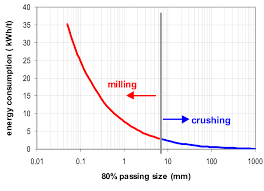The image above represents energy used in comminution.

To compute for energy used in comminution, three essential parameters are needed and these parameters are Constant (KR), Surface Area of Product (Ap) and Surface Area of Feed (Af).

The formula for calculating the energy used in comminution:

E = KR(Ap – Af)

Where:

E = Energy used in Comminution
KR = Constant
Ap = Surface Area of Product
Af = Surface Area of Feed

Let’s solve an example;
Find the energy used in comminution when the constant is 32, the surface area of product is 8 and the surface area of feed is 4.

This implies that;

KR = Constant = 32
Ap = Surface Area of Product = 8
Af = Surface Area of Feed = 4

E = KR(Ap – Af)
E = 32(8 – 4)
E = 32(4)
E = 128

Therefore, the energy used in comminution is 128 J.

Calculating the Constant when the Energy used in Comminution, the Surface Area of Product and the Surface Area of Feed is Given.

KR = E / (Ap – Af)

Where:

KR = Constant
E = Energy used in Comminution
Ap = Surface Area of Product
Af = Surface Area of Feed

Let’s solve an example;
Find the constant when the energy used in comminution is 24, the surface area of product is 15 and the surface area of feed is 7.

This implies that;

E = Energy used in Comminution = 24
Ap = Surface Area of Product = 15
Af = Surface Area of Feed = 7

KR = E / (Ap – Af)
KR = 24 / (15 – 7)
KR = 24 / 8
KR = 3

Therefore, the constant is 3.

## How to Calculate and Solve for Energy Input | Rittinger’s Equation of Comminution | Mineral Processing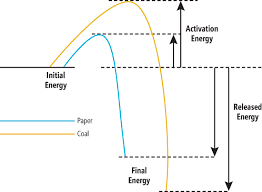The image above represents energy input.

To compute for energy input, three essential parameters are needed and these parameters are Constant (K), Feed Size (D1) and Product Size (D2).

The formula for calculating energy input:

E = K(1/D21/D1)

Where:

E = Energy Input
K = Constant
D1 = Feed Size
D2 = Product Size

Let’s solve an example;
Find the energy input when the constant is 10, the feed size is 2 and the product size is 8.

This implies that;

K = Constant = 10
D1 = Feed Size = 2
D2 = Product Size = 8

E = K(1/D21/D1)
E = 10(1/81/2)
E = 10(0.125 – 0.5)
E = 10(-0.375)
E = -3.75

Therefore, the energy input is -3.75 J.

## How to Calculate and Solve for Degree of Locking | Mineral Processing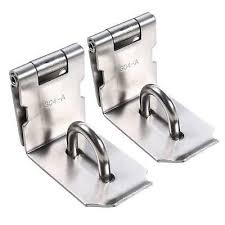The image above represents degree of locking.

To compute for degree of locking, two essential parameters are needed and these parameters are Free Particles (m) and Locked Particles (n).

The formula for calculating degree of locking:

%L = 100 (n / m + n)

Where:

%L = Degree of Locking
m = Free Particles
n = Locked Particles

Let’s solve an example;
Find the degree of locking when the free particles is 10 and the locked particles is 5.

This implies that;

m = Free Particles = 10
n = Locked Particles = 5

%L = 100 (n / m + n)
%L = 100 (5 / 10 + 5)
%L = 100 (5 / 15)
%L = 100 (0.33)
%L = 33.33

Therefore, the degree of locking is 33.33%.

## How to Calculate and Solve for Degree of Liberation | Mineral Processing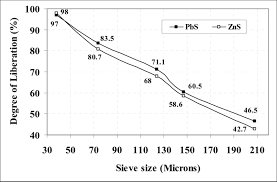The image above represents degree of liberation.

To compute for degree of liberation, two essential parameters are needed and these parameters are Free Particles (m) and Locked Particles (n).

The formula for calculating degree of liberation:

%F = 100 (m / m + n)

Where:

%F = Degree of Liberation
m = Free Particles
n = Locked Particles

Let’s solve an example;
Find the degree of liberation when the free particles is 21 and the locked particles is 7.

This implies that;

m = Free Particles = 21
n = Locked Particles = 7

%F = 100 (m / m + n)
%F = 100 (21 / 21 + 7)
%F = 100 (21 / 28)
%F = 100 (0.75)
%F = 75

Therefore, the degree of liberation is 75%.

## How to Calculate and Solve for Average Velocity | Tube-Bundle Theory | Transport Phenomena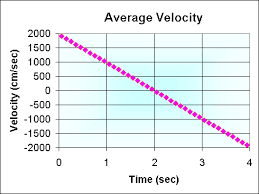The image above represents average velocity.

To compute for average velocity, five essential parameters are needed and these parameters are Constant of Proportionality (k1), Change in Pressure (ΔP), Hydraulic Radius (Rh), Length of Pipe (L) and Viscosity (η).

The formula for calculating average velocity:

v = k1ΔPRh² /

Where:

v = Average Velocity | Tube-Bundle Theory
k1 = Constant of Proportionality
ΔP = Change in Pressure
L = Length of Pipe
η = Viscosity

Let’s solve an example;
Find the average velocity when the constant of proportionality is 10, the change in pressure is 5, the hydraulic radius is 2, the length of pipe is 4 and the viscosity is 8.

This implies that;

k1 = Constant of Proportionality = 10
ΔP = Change in Pressure = 5
Rh = Hydraulic Radius = 2
L = Length of Pipe = 4
η = Viscosity = 8

v = k1ΔPRh² /
v = (10)(5)(2)² / (4)(8)
v = (10)(5)(4) / 32
v = 200 / 32
v = 6.25

Therefore, the average velocity is 6.25 m/s.